#### 2023-06-08

This vignette will explore some of the more advanced mapping features of usmap. Before continuing, be sure to check out Mapping the US as that will cover more of the basics of plotting US maps and styling them with ggplot2.

## Labels

As of usmap 0.4.0, maps with state labels can be created:

usmap::plot_usmap("states", labels = TRUE)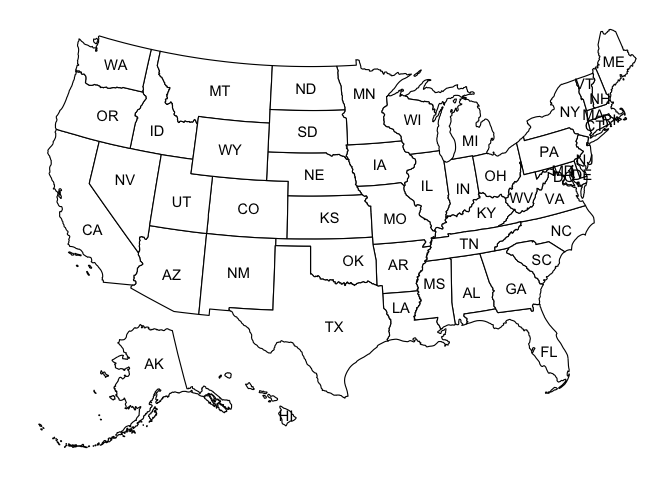usmap 0.5.0 adds the ability to add county labels:

usmap::plot_usmap("counties", include = c("MA", "CT", "RI"), labels = TRUE)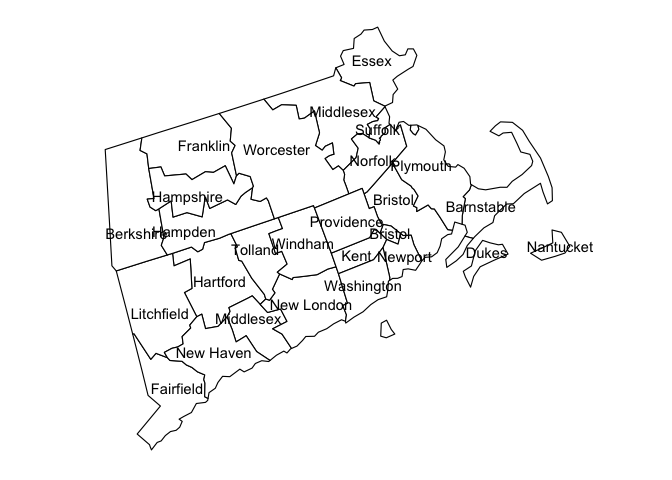Labels can be colored using the label_color parameter:

usmap::plot_usmap("counties",
include = c("MA", "CT", "RI"),
labels = TRUE, label_color = "blue")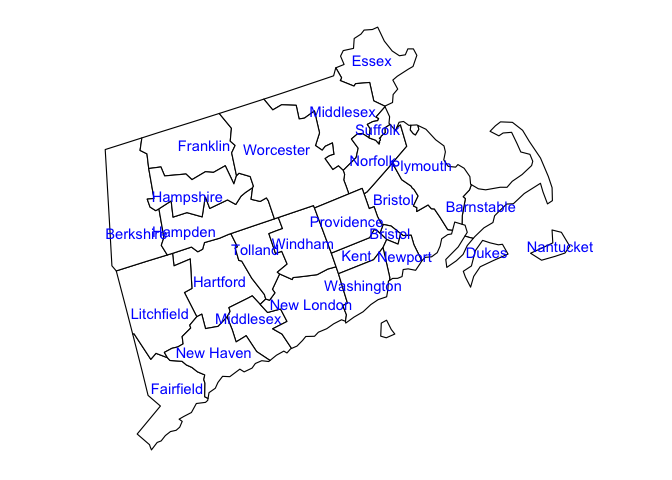## ggplot2 aesthetic mapping parameters

Parameters used by the map’s aesthetic mapping (ggplot2::aes) can be passed directly via plot_usmap by adding the parameters anywhere at the call site:

usmap::plot_usmap("counties",
include = c("MA", "CT", "RI"),
labels = TRUE, label_color = "blue",
fill = "yellow", alpha = 0.25, color = "orange", linewidth = 2)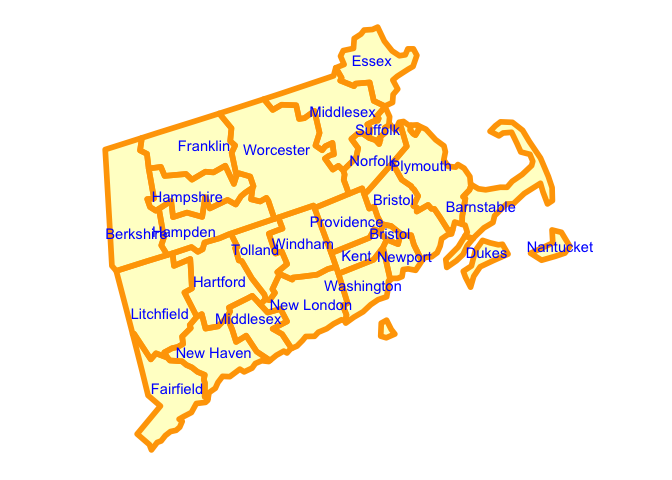Notice in this case we set the fill and alpha parameters to fill in the counties with a semi-transparent yellow color.

The following parameters are supported:

• fill: fill color of the state/county polygons
• alpha: transparency of the state/county polygon fill colors
• color/colour: line color of the state/county polygons
• linewidth: thickness of the state/county polygon lines

## Transform data frames to match usmap projection

Data sets with longitude and latitude coordinates can be transformed to match the projection used in usmap (Albers Equal Area projection). This is convenient for plotting location-specific data and values using ggplot2 layers such as geom_point and geom_label.

#### Projection

The projection used by usmap can also be accessed by using usmap_crs():

usmap::usmap_crs()@projargs
#> The legacy packages maptools, rgdal, and rgeos, underpinning this package
#> will retire shortly. Please refer to R-spatial evolution reports on
#> https://r-spatial.org/r/2023/05/15/evolution4.html for details.
#> This package is now running under evolution status 0
#>  "+proj=laea +lat_0=45 +lon_0=-100 +x_0=0 +y_0=0 +ellps=sphere +units=m +no_defs"

A convenience method called usmap_transform is provided that transforms a data.frame containing longitude/latitude columns to use this projection. (Currently, only data.frames are supported. Other structures may be supported in the future.)

#### Example: earthquakes

Here is an example using the provided earthquakes data set:

library(usmap)
library(ggplot2)

eq_transformed <- usmap_transform(earthquakes)

plot_usmap() +
geom_point(data = eq_transformed, aes(x = x, y = y, size = mag),
color = "red", alpha = 0.25) +
labs(title = "US Earthquakes",
subtitle = "Source: USGS, Jan 1 to Jun 30 2019",
size = "Magnitude") +
theme(legend.position = "right")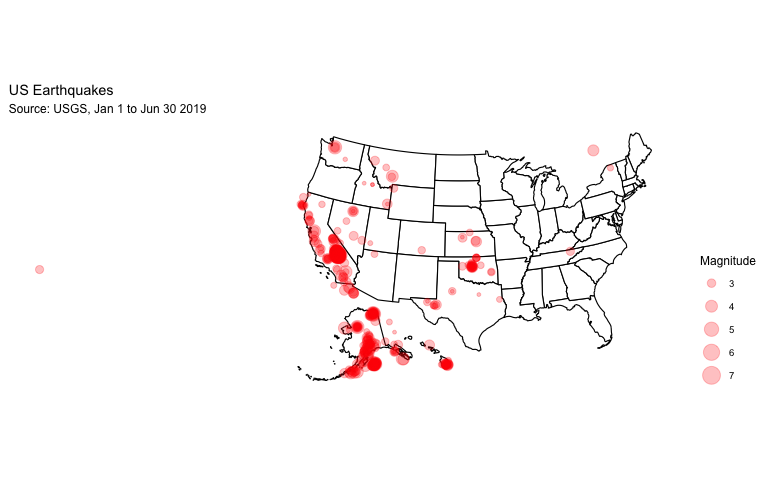#### Example: most populous city in each state

And a more comprehensive example using the provided citypop dataset:

library(usmap)
library(ggplot2)

cities_t <- usmap_transform(citypop)

plot_usmap(fill = "yellow", alpha = 0.25) +
ggrepel::geom_label_repel(data = cities_t,
aes(x = x, y = y, label = most_populous_city),
size = 3, alpha = 0.8,
label.r = unit(0.5, "lines"), label.size = 0.5,
segment.color = "red", segment.size = 1,
seed = 1002) +
geom_point(data = cities_t,
aes(x = x, y = y, size = city_pop),
color = "purple", alpha = 0.5) +
scale_size_continuous(range = c(1, 16),
label = scales::comma) +
labs(title = "Most Populous City in Each US State",
subtitle = "Source: US Census 2010",
size = "City Population") +
theme(legend.position = "right")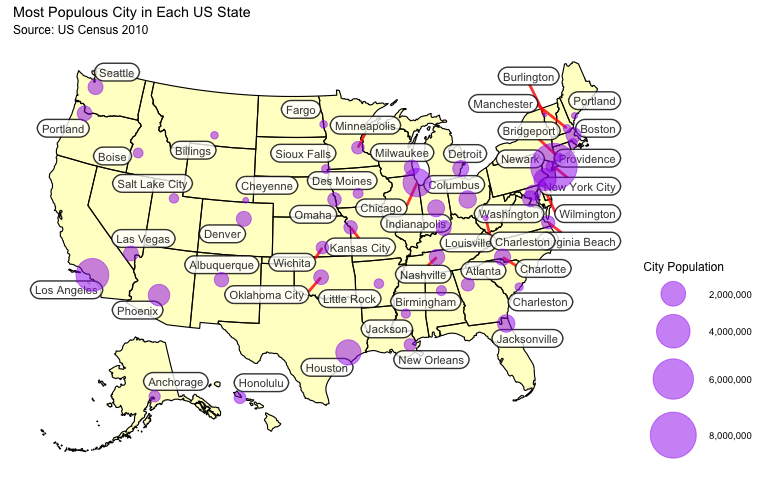The usmap_transform function, combined with the power of ggplot2 layers can allow for some very unique and complex data visualizations on the US map. The usmap_transform function also handles transforming points in the Alaska/Hawaii area so that they are appropriately displayed on their respective states.

Currently, usmap_transform does not trim any points that fall outside the Alaska/Hawaii/US bounding boxes so it is important to prepare the data beforehand by eliminating any points that should not be displayed on the map otherwise it could have undesirable results.